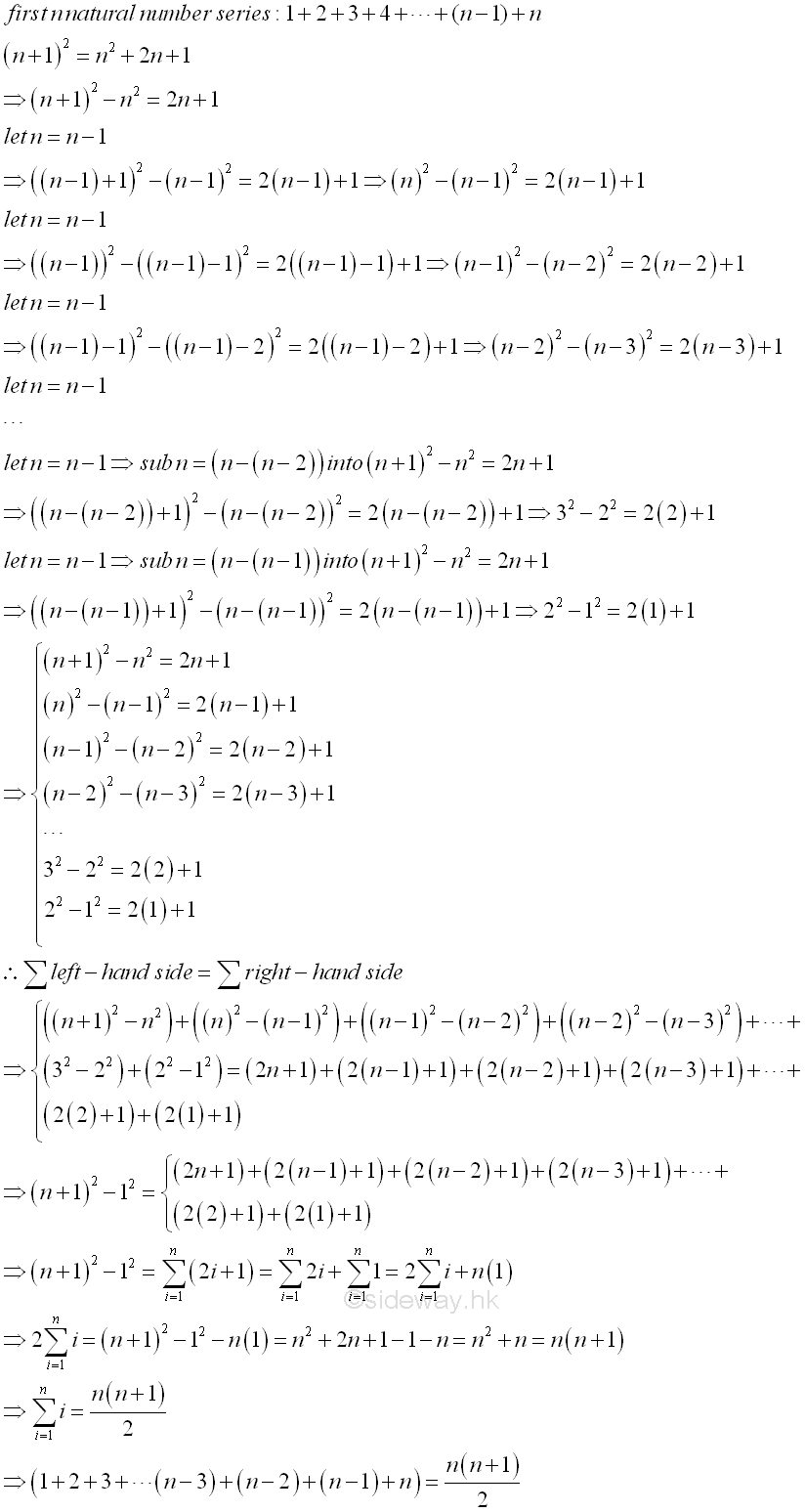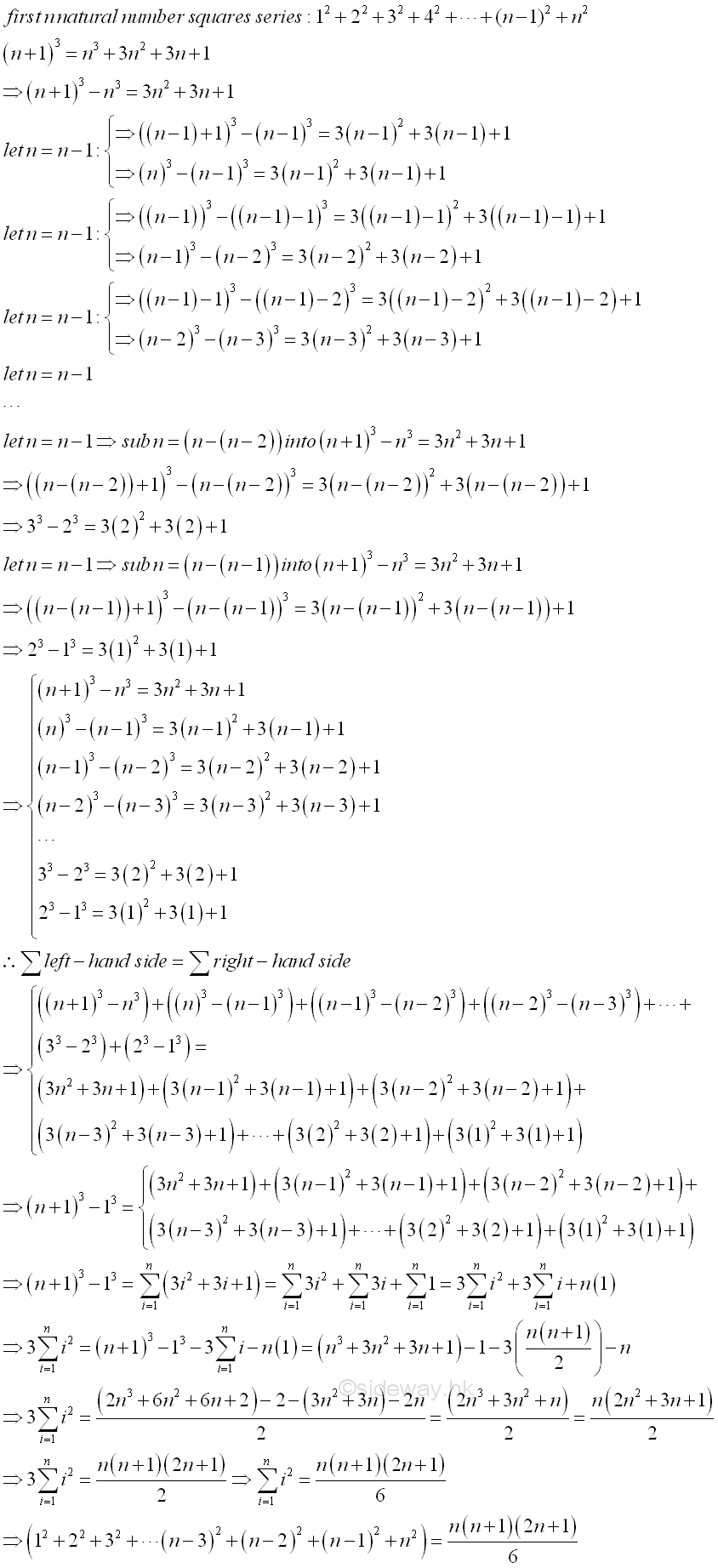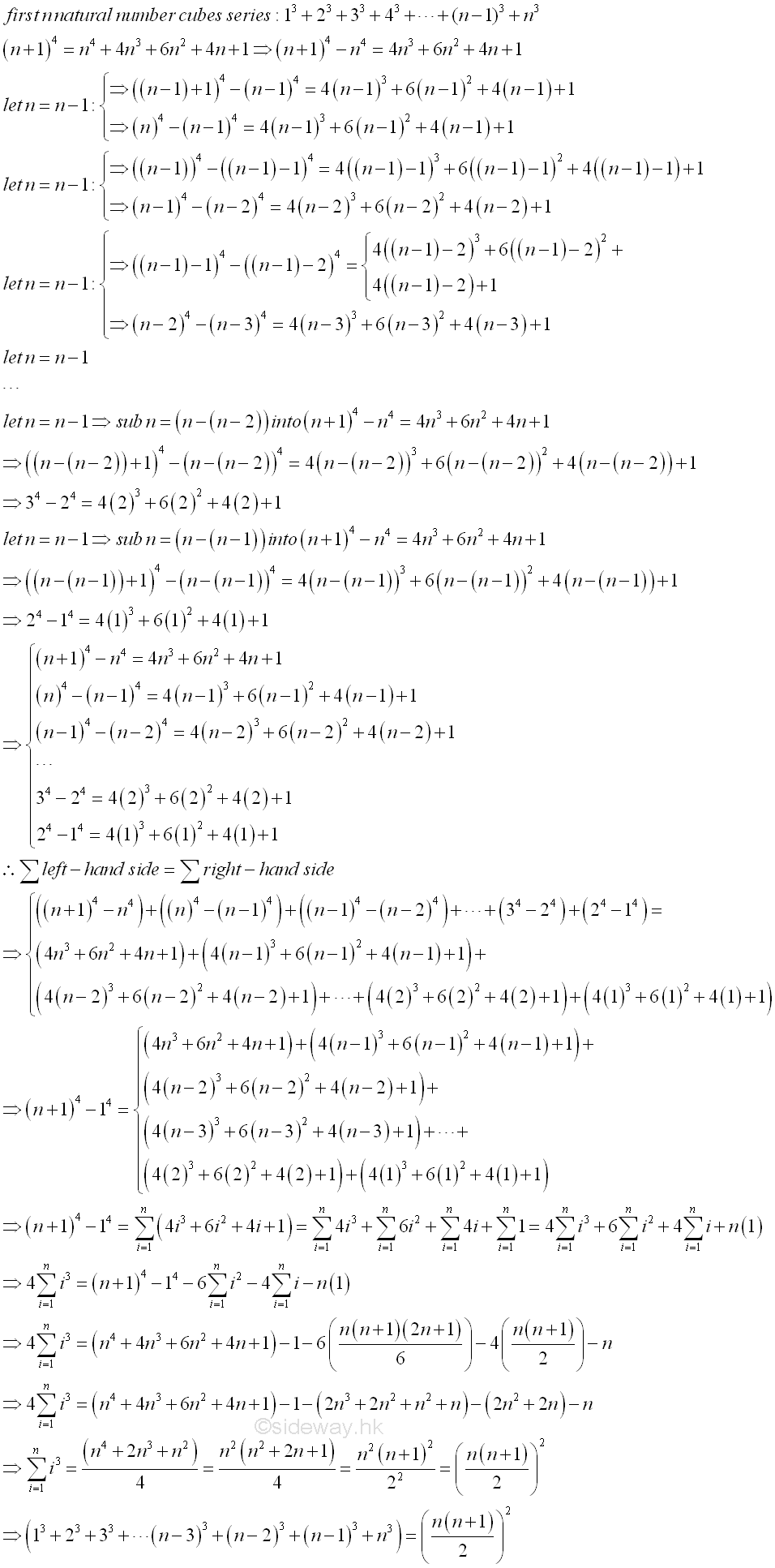output.to from Sideway
Algebra

Draft for Information Only

# Content

`  Powers of Natural Numbers Series   First n Natural Numbers Series   First n Natural Number Squares Series   First n Natural Number Cubes Series`

## Powers of Natural Numbers Series

Powers of natural numbers series can also be found algebraically by making use of the power of a binomial.

### First n Natural Numbers Series

For the first n natural number series, 1+2+3+...+n, a binomial to the power of 2 can be used. By making use of the identity  (n+1)2=n2+2n+1⇒(n+1)2-n2=2n+1. Let n=n-1 and substitute into the identity, then (n)2-(n-1)2=2(n-1)+1. By repeating the n=n-1 substitution, and get (n-1)2-(n-2)2=2(n-2)+1. By repeating the n=n-1 substitution again, and get (n-2)2-(n-3)2=2(n-32=2(n-3)+1. By continuing the n=n-1 substitution process until the original variable n is reduced to 1, that is ..., ((n-(n-2))+1)2-(n-(n-2))2=2(n-(n-2))+1, (1+1)2-(1)2=2(1)+1,  a totally n sets of identities are obtained. By adding up all the identities, the left hand side is reduced to the first and last term, (n+1)2-12, while the right hand side remains the summation of all terms. After rearranging the similar terms on the right hand, the identity becomes (n+1)2-12=2(n+(n-1)+(n-2)+(n-3)+...+2+1)+n(1). Imply (n+(n-1)+(n-2)+(n-3)+...+2+1)=(((n+1)2-1)-n)/2=(n2+n)/2=n(n+1)/2. Imply### First n Natural Number Squares Series

For the first n natural number squares series, 12+22+32+...+n2, a binomial to the power of 3 can be used. By making use of the identity  (n+1)3=n 3+ 3n2+3n+1⇒(n+1)3-n3=3n2+3n+1. Let n=n-1 and substitute into the identity, then (n)3-(n-1)3=3(n-1)2+3(n-1)+1. By repeating the n=n-1 substitution, and get (n-1)3-(n-2)3=3(n-2)2+3(n-2)+1. By repeating the n=n-1 substitution again, and get (n-2)3-(n-3)3=3(n-3)2+3(n-3)+1. By continuing the n=n-1 substitution process until the original variable n is reduced to 1, that is ..., (n-(n-2)+1)3-(n-(n-2))3=3(n-(n-2))2+3(n-(n-2))+1, (1+1)3-(1)3=3(1)2+3(1)+1,  a totally n sets of identities are obtained. By adding up all the identities, the left hand side is reduced to the first and last term, (n+1)3-13, while the right hand side remains the summation of all terms. After rearranging the similar terms on the right hand, the identity becomes (n+1)3-13=3(n2+(n-1)2+(n-2)2+(n-3)2+...+22+12)+3(n+(n-1)+(n-2)+(n-3)+...+2+1)+n(1). Imply (n2+(n-1)2+(n-2)2+(n-3)2+...+22+12) =((n+1)3-1-3(n+(n-1)+(n-2)+(n-3)+...+2+1)-n)/3=(n3+3n2+3n+1-1-n)/3-(n+(n-1)+(n-2)+(n-3)+...+2+1) =(n3+3n2+2n)/3-n(n+1)/2 =(2(n3+3n2+2n)-3(n2+n))/6 =(2n3+3n2+n)/6=(n(2n2+3n+1))/6=(n(n+1)(2n+1))/6. Imply### First n Natural Number Cubes Series

For the first n natural number cubes series, 13+23+33+...+n3, a binomial to the power of 4 can be used. By making use of the identity  (n+1)4=n 4+4n3+6n2+4n+1⇒(n+1)4-n4= 4n3+6n2+4n+1. Let n=n-1 and substitute into the identity, then (n)4-(n-1)4= 4( n-1) 3+6 ( n-1) 2+4 (n-1)+1. By repeating the n=n-1 substitution, and get (n-1)4-(n-2)4= 4 (n-2) 3+6 (n-2) 2+4 ( n-2)+1. By repeating the n=n-1 substitution again, and get (n-2)4-(n-3)4= 4 ( n-3) 3+6 (n-3) 2+4 ( n-3)+1. By continuing the n=n-1 substitution process until the original variable n is reduced to 1, that is ..., (n-(n-2)+1)4-(n-(n-2))4= 4(n-(n-2))3+6(n-(n-2))2+4(n-(n-2))+1, (1+1)4-(1)4=4 (1)3+6(1)2+4(1)+1,  a totally n sets of identities are obtained. By adding up all the identities, the left hand side is reduced to the first and last term, (n+1)4-14, while the right hand side remains the summation of all terms. After rearranging the similar terms on the right hand, the identity becomes (n+1)4-14= 4(n3+(n-1)3+(n-2)3+(n-3)3+...+23+13)+6(n2+(n-1)2+(n-2)2+(n-3)2+...+22+12)+4(n+(n-1)+(n-2)+(n-3)+...+2+1)+n(1). Imply (n3+(n-1)3+(n-2)3+(n-3)3+...+23+13) =((n+1)4-1-6(n2+(n-1)2+(n-2)2+(n-3)2+...+22+12)-4(n+(n-1)+(n-2)+(n-3)+...+2+1)-n)/4=(n 4+4n3+6n2+4n+1-1-n)/4-6(n2+(n-1)2+(n-2)2+(n-3)2+...+22+12)/4-(n+(n-1)+(n-2)+(n-3)+...+2+1) =(n 4+4n3+6n2+3n)/4-n(n+1)(2n+1)/4-n(n+1)/2 =((n4+4n3+6n2+3n)- (2n3+2n 2+ n2+n) -2(n2+n))/4 =( n4+2n3+n2)/4=(n 2(n2+2n+1))/4=(n 2(n+1) 2)/4=((n(n+1))/2) 2. Imply©sidewayReferences

1. B. Joseph, 1978, University Mathematics: A Textbook for Students of Science & Engineering, Blackie & Son Limited, HongKong
2. Wheatstone, C., 1854, On the Formation of Powers from Arithmetical Progressions, Proceedings of The Royal Society of London, Vol 7, p145-151,, London
3. Stroud, K.A., 2001, Engineering Mathematics, Industrial Press, Inc, NY
4. Coolidge, J.L., 1949, The Story of The Binomial Theorem, The American Mathematical Monthly, Vol 56, No.3, Mar, pp147-157ID: 130500021 Last Updated: 2013/5/21 Revision: 0 Ref:Home (5)

Business

Management

HBR (3)

Information

Recreation

Hobbies (7)

Culture

Chinese (1097)

English (336)

Reference (66)

Computer

Hardware (149)

Software

Application (187)

Digitization (24)

Numeric (19)

Programming

Web (648)CSS (SC)

ASP.NET (SC)

HTML

Knowledge Base

Common Color (SC)

Html 401 Special (SC)

OS (389)

MS Windows

Windows10 (SC)

.NET Framework (SC)

DeskTop (7)

Knowledge

Mathematics

Formulas (8)

Number Theory (206)

Algebra (20)

Trigonometry (18)

Geometry (18)

Calculus (67)

Complex Analysis (21)

Engineering

Tables (8)

Mechanical

Mechanics (1)

Rigid Bodies

Statics (92)

Dynamics (37)

Fluid (5)

Control

Acoustics (19)

Biology (1)

Geography (1)

Copyright © 2000-2019 Sideway . All rights reserved Disclaimers last modified on 10 Feb 2019